×

Search anything:

# Dynamic Mode Decomposition (DMD): An Overview of the Mathematical Technique and Its Applications

#### Machine Learning (ML)### What is DMD?

Dynamic Mode Decomposition (DMD) is a data-driven method used to analyze and extract dynamic behavior from high-dimensional data sets. It is a powerful tool for studying fluid dynamics, image processing, and other complex systems. DMD is based on the Singular Value Decomposition (SVD) of a data matrix, and it provides a low-dimensional approximation of the original system.

This article will explain the key concepts behind DMD, including SVD and other related techniques, and provide examples of how it can be used in different applications.

### Introduction

The study of complex systems often requires the analysis of high-dimensional data. For example, in fluid dynamics, researchers may collect data on the velocity field of a fluid over time. This data is typically represented as a matrix, where the rows correspond to spatial locations and the columns correspond to time steps. However, analyzing such high-dimensional data sets is challenging. One approach is to use DMD, which extracts low-dimensional models of the data by identifying the underlying dynamic behavior. This technique can be used to extract coherent spatiotemporal patterns from noisy or incomplete data, and it can provide insight into the underlying dynamics of a system. DMD has been successfully applied in various fields, including fluid dynamics, neuroscience, and epidemiology, among others.

Now, let's see the mathematics behind it.

### Mathematics

Assume that X1 captured at time t and X2 is captured at time t + ∆t.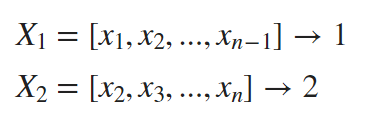DMD computes the leading eigendecomposition of the linear operator which maps to these two matrices X1 and X2 as given below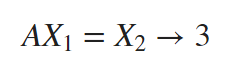Now, to calculate the best fit value for the linear operator A, the pseudo inverse of X1 is taken as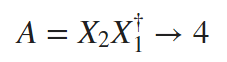where † is the pseudo-inverse operation. But, in a real-world scenario, n can take a very large dimension and hence A will be much larger to compute. Therefore this does not seem to be an optimal solution to proceed further. Thus we need a smaller matrix that would be easy and comfortable to compute and it should also capture the maximum possible characteristics of A.To obtain the approximation of A with the lower dimensions (named as A'), the number of modes for the analyses of data matrices is truncated by DMD.

In the first step of the DMD algorithm, the SVD of X1 is computed as follows.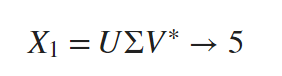Taking the inverse on both sides results as follows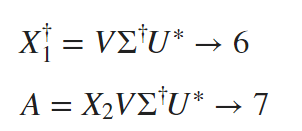Equation 7 is obtained from 4 and 6.

The dimension of A is relatively high. This leads to the involvement of Proper Orthogonal Decomposition (POD). This is a statistical method that helps in obtaining low-dimensional abstraction for high-dimensional data. Hence, we produce A' for ease of computation. Here, A and A' are similar matrices. Both the matrices will have the same eigenvalues and also the same number of eigenvectors. With this information, it is possible to compute the similarity transformation matrix A' as follows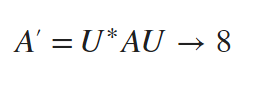We can prove that both A and A' have same eigen values by taking the eigendecomposition of A',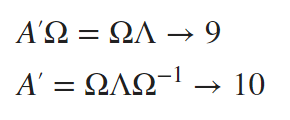From equation 8 and 10,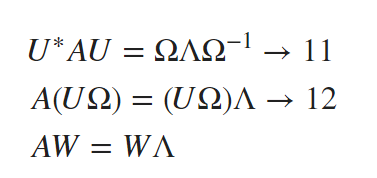W contains (n−1) columns where all of them represent the eigenvectors of A and Λ is the diagonal matrix with (n−1) entries which are the eigenvalues of A.

### Applications

Applications of it, for instance:

Fluid Dynamics: In order to extract coherent structures from flow fields, such as vortices and eddies, and to examine their temporal and spatial evolution, DMD is frequently employed in fluid dynamics.

Aerospace: DMD is used in the aerospace sector to evaluate and improve the aerodynamics of aeroplanes and spacecraft, including the development of wing forms and control surfaces.

Medical Imaging: DMD can be used to examine MRI scan data and other types of medical imaging data to look for patterns that might point to disease.

Robotics: To recognise and follow the motion of objects, forecast their future motion, and enhance control methods, DMD is used in robotics.

Machine Learning: DMD can be used as a pre-processing step for machine learning algorithms to extract significant features from high-dimensional data, lowering the complexity of the input data and enhancing the precision of the machine learning model.

Climate research: DMD is used in climate research to analyse the data and spot patterns and trends. The temporal variations in different spatial points can be correlated with DMD.

### Conclusion

In conclusion of this article at OpenGenus, Dynamic Mode Decomposition (DMD) has become a potent mathematical tool with a variety of uses in numerous disciplines. By locating underlying patterns and dynamics, DMD offers insightful information into complex systems, from fluid dynamics and structural vibrations to neuroscience and data processing. DMD is anticipated to play an increasingly significant role in expanding our understanding of complex systems and contributing to a variety of practical applications as a result of continuous developments in computational techniques and machine learning. It is obvious that DMD has the potential to fundamentally alter how we approach and resolve complicated challenges in a variety of domains as research in this area continues to advance.

## Question

#### How does the proper orthogonal decomposition (POD) contribute to the use of Dynamic Mode Decomposition (DMD)?

It reduces the dimensionality of the data
It provides an initial condition for DMD
It increases the accuracy of the DMD results
It replaces DMD in the analysis of complex systems
Proper Orthogonal Decomposition (POD) identifies the most significant patterns in the data and generates a reduced-order model that captures the essential features of the system.